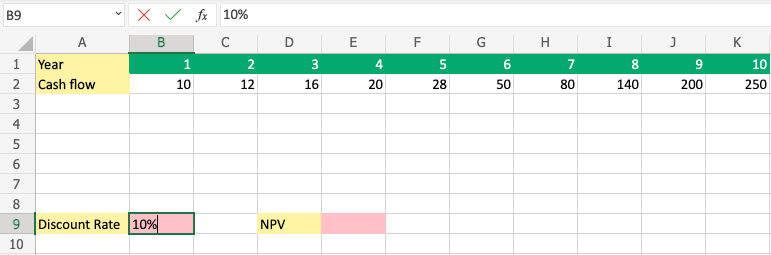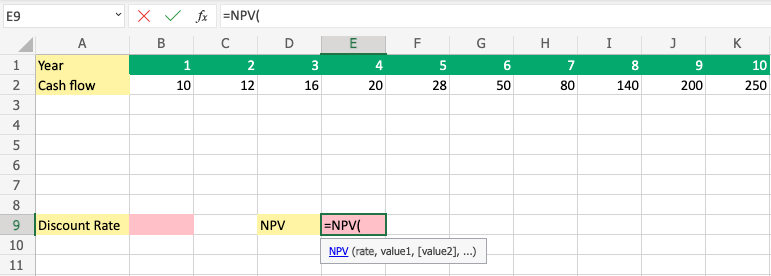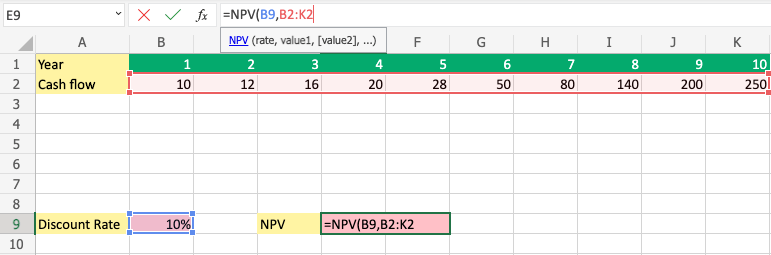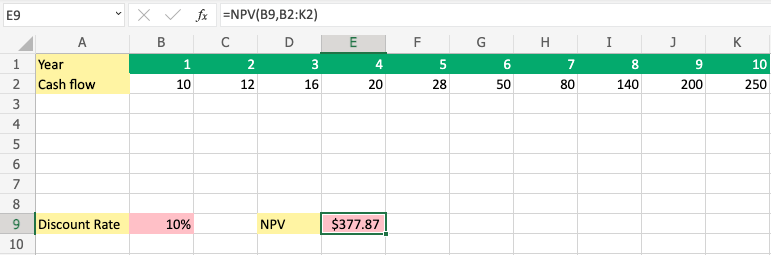# Excel NPV Function

## NPV Function

The NPV function is used to calculate the Net Present Value (NPV)

It is typed `=NPV`

=NPV(rate, value1, value2, ...)

rate: The Discount rate.

value: The cells where the cash flows are.

## How to Use NPV Excel Function

To calculate and find out whether an investment is positive in the future, use NPV.

##### Step 1) Type the rate value

The rate is the required Discount Rate that you want to use.

We will use `10%` in this example.

1. Select cell (`B9`)
2. Type `10%`
3. Hit enter

Ensure that you enter the value as percentage(%).##### Step 2) Start the NPV function
1. Select a cell (`E9`)
2. Type `=NPV`
3. Double click the NPV commandCopy Values
##### Follow along the tutorial by trying it yourself!

Copy the values in the example above and try it on your own!##### Step 3) Add values to the function

Let us add the rate and the values from 1 to 10 to the function.

1. Select cell (`B9`)
2. Type `,` or `;`
3. Select the range (`B2:K2`)
4. Hit enter

Note: The different parts of the function are separated by a symbol, like comma `,` or semicolon `;`

The symbol depends on your Language Settings.Did you calculate 377,87?

Congratulations! you have just calculated the NPV for ten years using a discount rate of 10%

W3Schools is optimized for learning and training. Examples might be simplified to improve reading and learning. Tutorials, references, and examples are constantly reviewed to avoid errors, but we cannot warrant full correctness of all content. While using W3Schools, you agree to have read and accepted our terms of use, cookie and privacy policy.